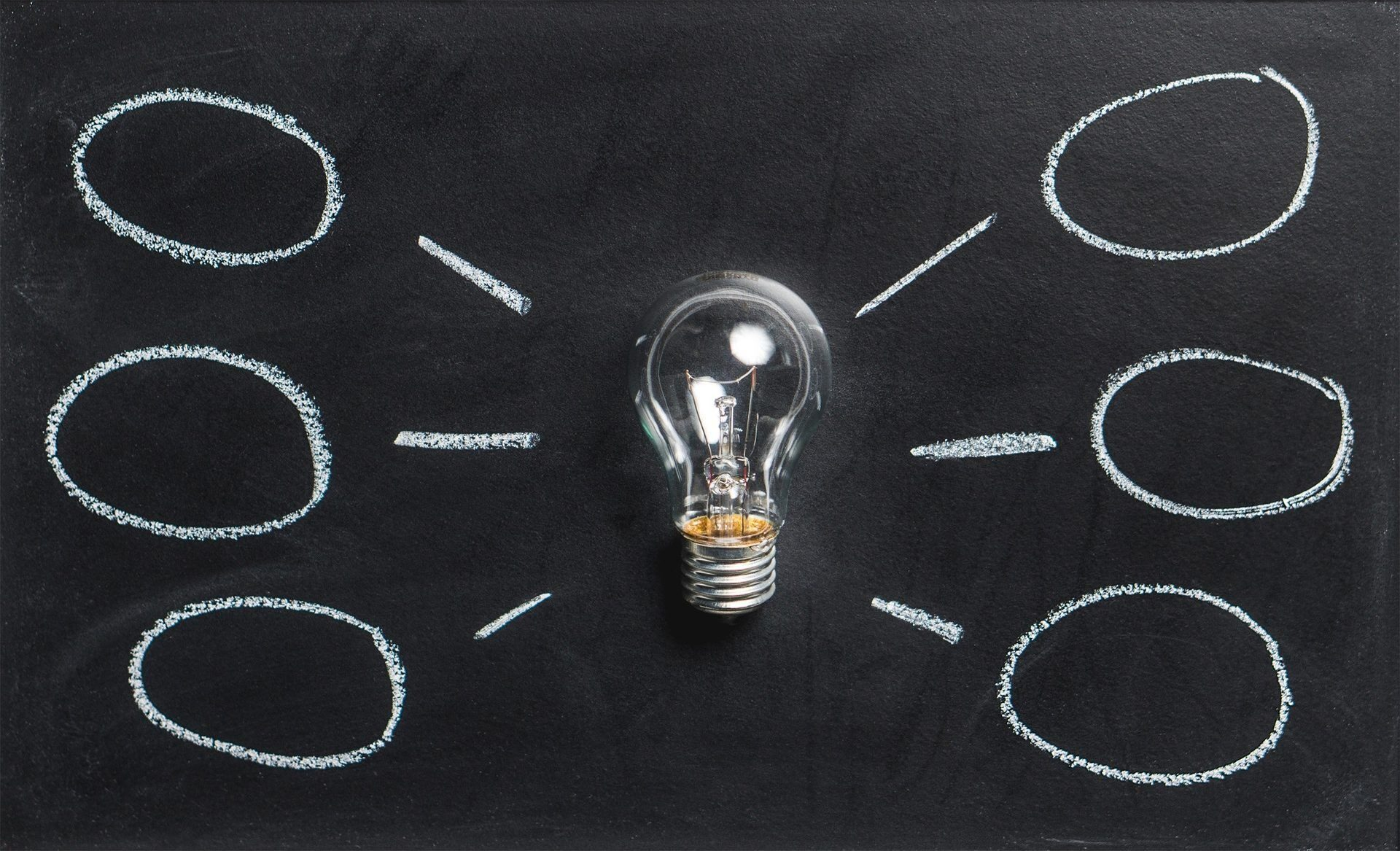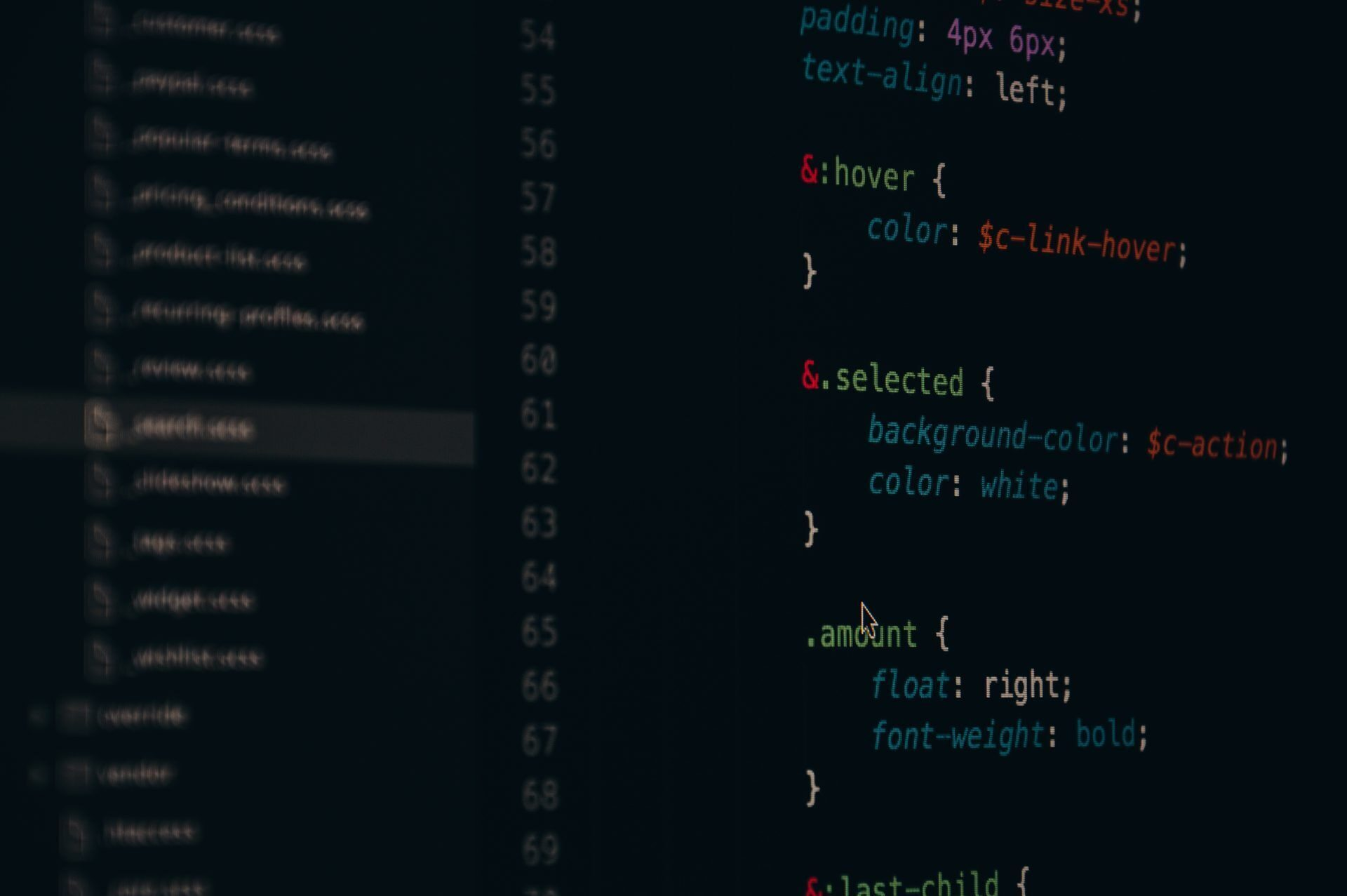What is an epoch in machine learning? An epoch in machine learning refers to one full pass of the training dataset through the algorithm whenever you wish to train a model with some data. As a result, it is a learning algorithm hyperparameter. With the rise of the digital age, many people have started looking for information on this rapidly evolving topic of machine learning.

According to Acumen Research and Consulting, the global deep learning market will reach 415 billion USD by 2030. Are you wondering about machine learning benefits for your business, but do these terms confuse you? Don’t worry; we have already explained what is an epoch in machine learning for you.

## What is an epoch in machine learning?

A complete cycle through the entire training dataset can be considered an epoch in machine learning, reflecting how many passes the algorithm has made throughout the training.

The number of epochs in training algorithms can reach thousands, and the procedure is designed to go on indefinitely until the model error is sufficiently reduced. Examples and tutorials frequently include 10, 100, 1000, or even greater numbers.A complete cycle through the entire training dataset can be considered an epoch in machine learning, reflecting how many passes the algorithm has made throughout the training

Advanced algorithms are used in machine learning to evaluate data, learn from it, and apply these learning points to find interesting patterns. Machine learning models are developed using many epochs. As this entails learning per what is learned from the dataset, some human interaction is necessary for the early stages.

There are two different categories of machine learning models: supervised learning models and unsupervised learning models. Specific datasets are needed for these models to build their learning ability, and these training datasets must be planned following the desired result and the task(s) that the agent will need to complete.

Check out the history of machine learning

When trying to fully define an epoch, which is primarily considered as one cycle of the entire training dataset, it is important to comprehend the fundamental concepts and terms that make up an epoch in this context. The aggregate of data batches and iterations that make up an epoch is ultimately what makes up an epoch.

Datasets are organized into batches (especially when the data is very large). One batch is run through the model and sometimes considered one iteration by those who misuse the phrase. Iteration and an epoch are typically used synonymously.

The number of epochs equals the number of iterations if the batch size is the entire training dataset. Generally speaking, this is not the case for practical reasons. Multiple epochs are often used while creating models. The general relationship would be de = ib when the dataset size is d, the number of epochs is e, the number of iterations is I, and the batch size is b.

For instance, if we define the “task” as getting from point A to point B, we may define each feasible path from point A to point B as an “epoch,” and the precise route information, such as stops and turns, as the “iterations.”

Are you confused? Let’s explore them separately.The number of training samples used in one iteration is referred to as the “batch size” in machine learning

## What is a batch size in machine learning?

The number of training samples used in one iteration is referred to as the “batch size” in machine learning. There are three possibilities for the batch size:

• Batch mode: The iteration and epoch values are equal since the batch size equals the complete dataset.
• Mini-batch mode: The overall dataset size is smaller than the batch size, which is more than one. Usually, a sum can be divided by the size of the entire dataset.
• Stochastic mode: Where there is a single batch size. As a result, the gradient and neural network parameters are changed following each sample.

### Batch size vs epoch in machine learning

• The batch size is the number of samples processed before the model changes.
• The quantity of complete iterations through the training dataset is the number of epochs.
• A batch must have a minimum size of one and a maximum size that is less than or equal to the number of samples in the training dataset.
• You can choose an integer value for the number of epochs between one and infinity. The process can be run indefinitely and even be stopped by criteria other than a predetermined number of epochs, such as a change (or lack thereof) in model error over time.
• They both have integer values and are hyperparameters for the learning algorithm, i.e., learning process parameters instead of internal model parameters discovered by the learning process.
• You must provide a learning algorithm’s batch size and the number of epochs.

To configure these parameters, there are no secret formulas. You must test many values to determine which ones solve your situation the best.A machine learning concept called an iteration denotes how many times the parameters of an algorithm are changed

## What is an iteration in machine learning?

A machine learning concept called an iteration denotes how many times the parameters of an algorithm are changed. The context will determine what this implies specifically. The following actions would typically be included in a single iteration of training a neural network:

• Batch processing of the training dataset.
• Calculating the cost function.
• Modification and backpropagation of all weighting factors.

### Epoch vs iteration in machine learning

An iteration entails the processing of one batch. All data is processed once within a single epoch.

For instance, if each iteration processes 10 images from a set of 1000 images with a batch size of 10, it will take 100 iterations to finish one epoch.

## How to choose the number of epochs?

The weights are changed after each iteration of the network, and the curve shifts from underfitting to ideal to overfitting. The number of epochs is a hyperparameter that must be decided before training starts, and there is no one-fit formula for choosing it.

### Does increasing epochs increase accuracy?

Whether working with neural networks or determining geologic timescales, more isn’t always better. You should find the best number for each case.

Check out the challenges of machine learning lifecycle management

## Why is the epoch important in machine learning?

Epoch is crucial in machine learning modeling because it helps identify the model that most accurately represents the data. The neural network must be trained using the supplied epoch and batch size.Since there are no established guidelines for choosing the values of either parameter, specifying them is more of an art than a science

Since there are no established guidelines for choosing the values of either parameter, specifying them is more of an art than a science. In reality, data analysts must test a variety of values before settling on one that solves a particular issue the best.

Monitoring learning performance by charting its values against the model’s error in what is known as a learning curve is one method of determining the appropriate epoch. These curves are highly helpful when determining if a model is overfitting, underfitting, or properly trained.

## How many epochs to train?

11 epochs are the ideal number to train most datasets.

It may not seem right that we must repeatedly run the same machine learning or neural network method after running the full dataset through it.

So it must be remembered that we employ gradient descent, an iterative process, to optimize learning. Therefore, updating the weights with just one pass or epoch is insufficient.The learning rate is a tuning parameter in an optimization method used in machine learning and statistics that choose the step size at each iteration while aiming to minimize a loss function

One epoch may also cause the model to become overfit.

## Learning rate in machine learning

The learning rate is a tuning parameter in an optimization method used in machine learning and statistics that choose the step size at each iteration while aiming to minimize a loss function.

Learning rate in machine learning figuratively depicts the rate at which a machine learning model “learns” because it determines how much newly obtained information supersedes previous knowledge. The term “gain” is frequently used in the literature on adaptive control to refer to the learning rate.

## Conclusion

Epoch is a term used in machine learning to describe how often the training data is run through the algorithm during all the data points.

A decent level of test data correctness may require hundreds to thousands of epochs due to real-world applications’ richness and variety of data.

Check out the real-life examples of machine learning